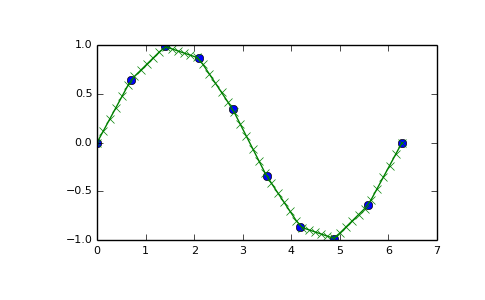# numpy.interp¶

numpy.interp(x, xp, fp, left=None, right=None)[source]

One-dimensional linear interpolation.

Returns the one-dimensional piecewise linear interpolant to a function with given values at discrete data-points.

Parameters: x : array_like The x-coordinates of the interpolated values. xp : 1-D sequence of floats The x-coordinates of the data points, must be increasing. fp : 1-D sequence of floats The y-coordinates of the data points, same length as xp. left : float, optional Value to return for x < xp, default is fp. right : float, optional Value to return for x > xp[-1], default is fp[-1]. y : {float, ndarray} The interpolated values, same shape as x. ValueError If xp and fp have different length

Notes

Does not check that the x-coordinate sequence xp is increasing. If xp is not increasing, the results are nonsense. A simple check for increasing is:

```np.all(np.diff(xp) > 0)
```

Examples

```>>> xp = [1, 2, 3]
>>> fp = [3, 2, 0]
>>> np.interp(2.5, xp, fp)
1.0
>>> np.interp([0, 1, 1.5, 2.72, 3.14], xp, fp)
array([ 3. ,  3. ,  2.5 ,  0.56,  0. ])
>>> UNDEF = -99.0
>>> np.interp(3.14, xp, fp, right=UNDEF)
-99.0
```

Plot an interpolant to the sine function:

```>>> x = np.linspace(0, 2*np.pi, 10)
>>> y = np.sin(x)
>>> xvals = np.linspace(0, 2*np.pi, 50)
>>> yinterp = np.interp(xvals, x, y)
>>> import matplotlib.pyplot as plt
>>> plt.plot(x, y, 'o')
[<matplotlib.lines.Line2D object at 0x...>]
>>> plt.plot(xvals, yinterp, '-x')
[<matplotlib.lines.Line2D object at 0x...>]
>>> plt.show()
```#### Previous topic

numpy.real_if_close

#### Next topic

Matrix library (numpy.matlib)